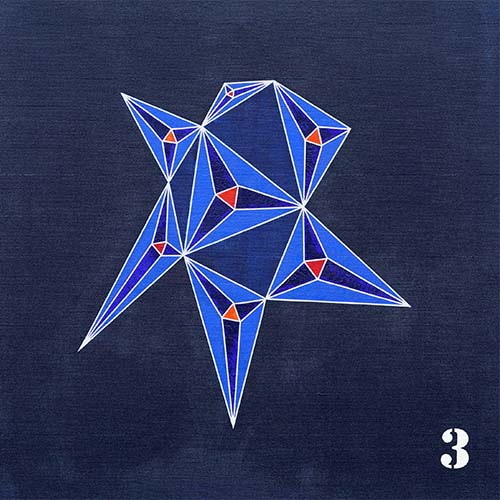### Morley's Miracle

Not all knowledge about triangles can be found as far back as in the writings of antiquity. Thus in 1899 the mathematician Frank Morley (1860 – 1937) discovered an astonishing result that had apparently been overlooked the ancient geometers:
In any triangle the angle trisectors lying near the sides intersect in an equilateral triangle.
His colleagues were so enthusiastic that they even spoke of Morley‘s Miracle. The proof itself is a bit challenging. But the beauty of Morley’s theorem lies in the simplicity of the statement.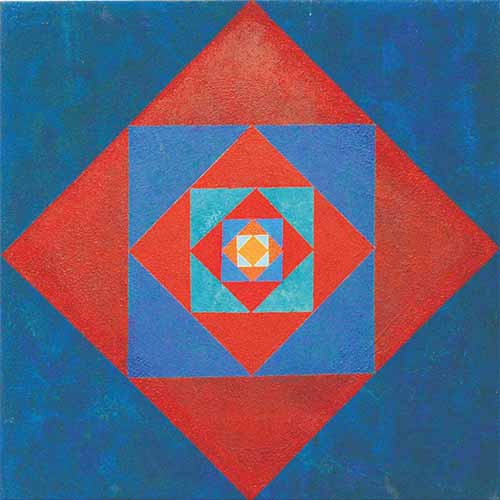### Infinity

"Infinity is a square without vertices."
Chinese saying
Try to explain the structure of the painting. How has Eugen Jost constructed the configuration?
In each step the area of a square is halved in comparison to the area of the preceding square.
The area of the biggest square equals 1. Determine the sum of the areas of this infinite number of squares.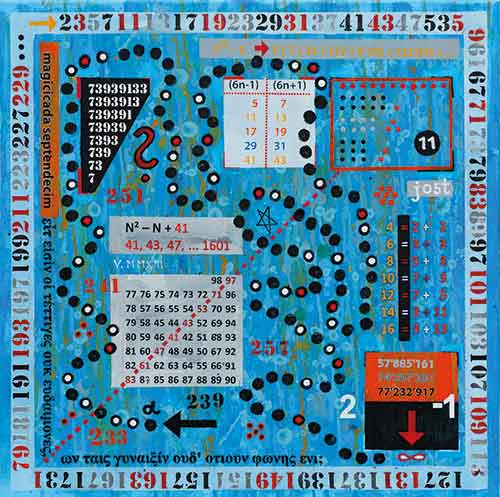### Prime Time

A prime number or prime is an integer greater than 1 that can be divided only by itself and 1.
A dot pattern runs through the painting like a winding snake. The individual dots symbolize the natural numbers (positive integers) in ascending order. The prime numbers 2, 3, 5, 7, 11, 13, 17, 19, … are especially marked by white spots.
The largest known prime 277 232 917 – 1 (effective May 2018) has 23 249 425 digits.### Mediterranean Geometry

“Geometry has two great treasures: one is the Theorem of Pythagoras; the other one the division of a line into extreme and mean ratio. The first may be compared to a measure of gold; the second may be named a precious jewel.”
Johannes Kepler (1571 – 1630)
Pythagoras of Samos (ca 570 BC – ca 500 BC)
Do you know the Theorem of Pythagoras?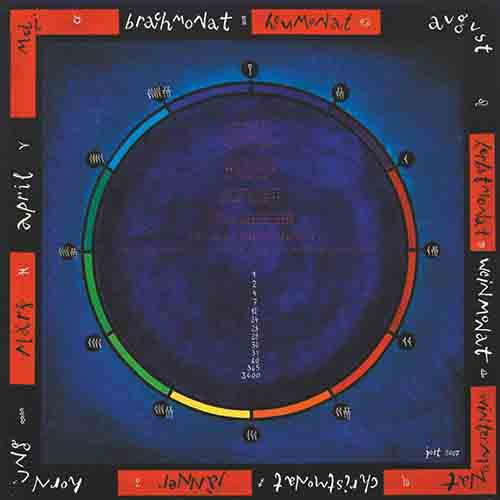### Spring, SUMER, Winter and Fall

Sumer in southern Mesopotamia is one of the earliest known civilizations in the world (the late ​6​th​ millennium to the early ​2​nd​ millennium BC).
The Mesopotamians used a sexagesimal (base 60) numeral system. This is the source of the current 60-minute hours and 24-hour days as well as the 360 degree circle.
A system based on 60 benefits from the great number of factors of the number 60.### A Walk with Mr. Euler

The Königsberg bridge problem asks if the seven bridges of the city of Königsberg, formerly in Germany but now known as Kaliningrad (part of Russia), over the river Pregel can all be traversed in a single trip without doubling back, with the additional requirement that the trip ends in the same place where it began.
This problem is equivalent to asking if the graph on four vertices and seven edges (cf Eugen Jost’s picture) has an Eulerian circuit. Euler’s answer was negative, and represented the beginning of graph theory.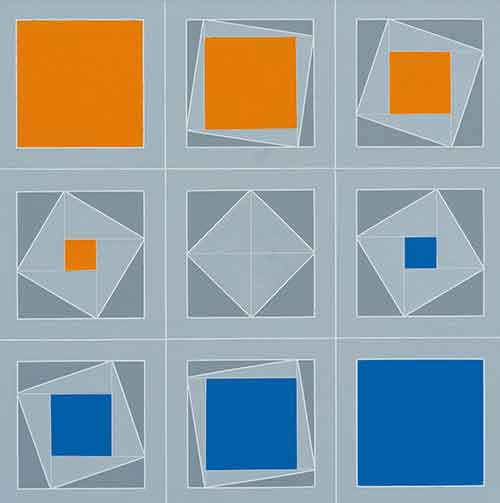### Dynamic Pythagoras

The picture sequence indicates a dynamic process where additional squares are generated in the interior of a fixed square.
Interesting questions arise: How do we get the interior squares? What connections to the Pythagorean theorem do exist?
Pythagorean Theorem:
In a right triangle the sum of the squares of the lengths of the legs equals the square of the length of the hypotenuse.### Hardy's Taxi

“A mathematician, like a painter or a poet, is a maker of patterns. If his patterns are more permanent than theirs, it is because they are made with ideas. ... The mathematician’s patterns, like the painter’s or poet’s, must be beautiful; the ideas, like the colours or the words, must fit together in a harmonious way. Beauty is the first test: there is no permanent place in the world for ugly mathematics. ...”
Godfrey Harold Hardy (1877 – 1947)### Girasole

Leonardo of Pisa (ca 1170 – ca 1240), also called Fibonacci, is known for the spreading of the Arabic numeral system in Central Europe, primarily through the publication of his Book of Calculation, the Liber Abaci.
How are the Fibonacci numbers 1, 1, 2, 3, 5, 8, 13, … generated?
Which Fibonacci number follows 987?
Find the connection between these numbers and the growth of a rabbit population.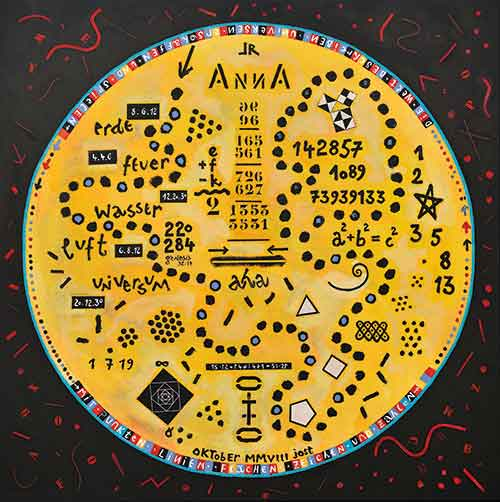### Symmetry

In everyday language symmetry refers to a sense of harmonious and beautiful proportion.
In mathematics symmetry has a more precise definition: A geometric object has symmetry if there is a transformation that maps the figure or object onto itself. There are several kinds of symmetry such as reflectional (mirror), rotational, translational symmetry, point reflection, and glide reflection.
A palindrome is a nice example for a special kind of symmetry. It is a word, number, or other sequence of characters that read equally backwards as forwards, such as otto or madam or racecar or 4884.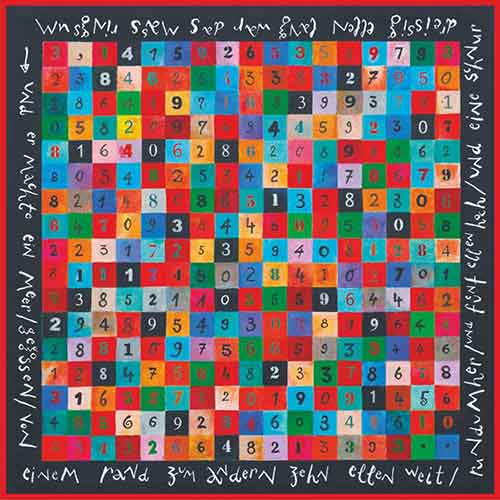### 3.14159...

π is defined as the ratio of the circumference of any circle to its diameter. It is an irrational number, which means that it cannot be expressed as a fraction ​ m/n ​, where m and n are integers. Consequently its decimal representation never ends or repeats. Beyond being irrational, π is a transcendental number. The picture shows 286 decimal places.
In the following sentence the number of letters in each word represents the successive digits of π: “How I want a drink, alcoholic of course, after the heavy lectures involving quantum mechanics.”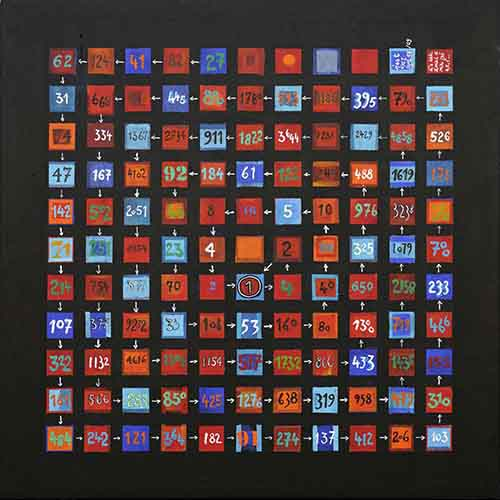### Thunderstorm

Start with any positive integer n: If n is an even number then halve it, or if it is an odd number then triple it and add 1, etc.
The conjecture is that this process will eventually reach the number 1, regardless of which positive integer is chosen initially.
The conjecture is named after Lothar Collatz (1910 – 1990), who introduced this idea in 1937.
This sequence is also known as hailstone sequence because the numbers usually undergo multiple descents and ascents like hailstones in a cloud.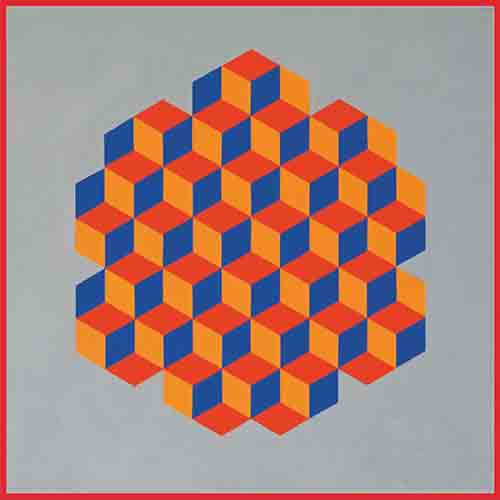### Squares, Rhombs, Cubes

In every civilization and culture coloured tilings and patterns appear among the earliest decorations. A tiling or tessellation of a plane is a collection of plane figures that fills the plane with no overlaps and no gaps.
Which geometrical objects do you discover in the picture?
In a regular polygon all interior angles are equal and all sides have the same length. Which regular polygon is hidden in the picture?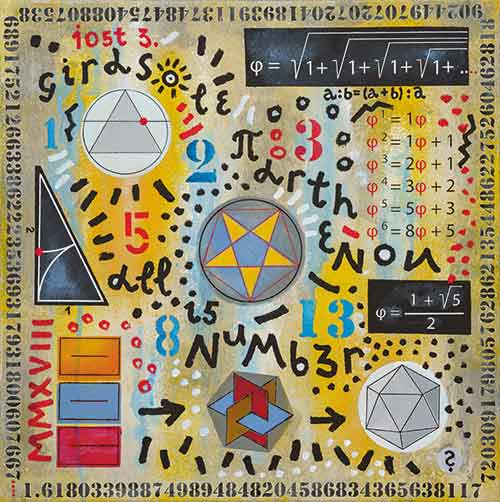### Golden Ratio

The golden ratio is a special number found by dividing a line segment into two parts so that the whole length divided by the longer part is equal to the longer part divided by the smaller part.
In other words:
The whole is to the section as the section is to the remainder.
The value of the golden ratio is an irrational number: φ = 1.6180339...### Magic Squares

A magic square of order n is normally composed of the positive integers 1, 2, 3, ..., n2. The magic of a magic square arises from the special arrangement of these n2 numbers in the square grid: The sum of the n numbers in each row, each column, and each diagonal is the same. The sum is called the magic constant.
Believe it or not: Each of the 25 squares of the painting contains a certain number. Sometimes more clearly, sometimes more encoded.
Describe some of the patterns, discover hidden numbers.